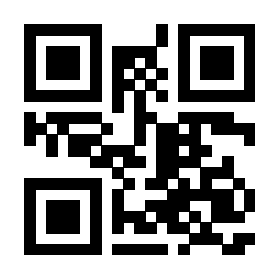5986
1 个回答tab页的切换并带动页面数据刷新这个是js控制的，所以从js入手跟踪调试了点击后的代码。

``````[0=>a,1=>b,2=>c]

{0=>a,1=>b,2=>c}

[a,b,c]

[0=>a,1=>b,-2=>c]

[1=>a,2=>b,3=>c]``````